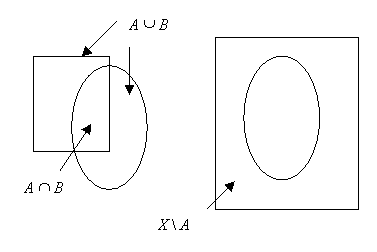EECS20N: Signals and Systems

Set Operations

• B A (B is a subset of A) if every element of B is an element of A. Equivalently A B (A is a superset of B)
• A B = {x | x A x ∈ B } -- intersection of A and B
• A B = {x | x A x ∈ B } -- union of A and B
• If A X , AC = X \ A = { x | x X, x A } -- complement of A in X